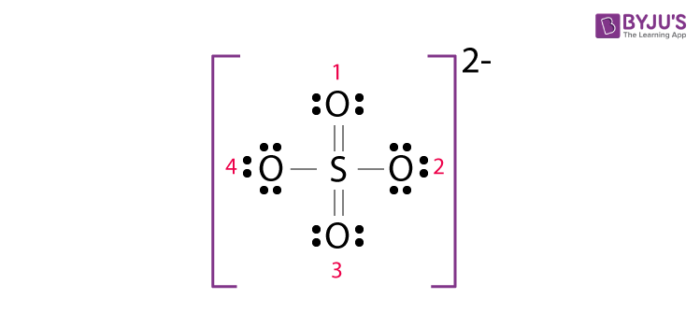# Formal Charge And Its Properties

### What is a Formal Charge?

A Formal charge is also known as a Fake Charge. It’s a theoretical charge over an individual atom of an ion as the real charge over a polyatomic molecule or ion is distributed on an ion as a whole and not over a single atom. The formal charge of an atom of a polyatomic molecule or ion is defined below.

### Formal Charge Definition:

“The formal charge over an atom of a polyatomic molecule or ion is the difference between the valence electron of that atom in the elemental state and the number of electrons assigned to that atom in Lewis structure.”

### Formal Charge Formula:

Mathematically, it can be expressed by following formula:

F.C. = [Total no. of valence e in free state] – [total no. of e assigned in Lewis structure] F.C. = [Total no. of valence e in free state] – [total no. of non-bonding pair e (lone pair)] – 1/2 [total no. of bonding e]

The factor of ½ is attached to the no. of bonding e because bonding e is shared between two atoms.

### Formula Charge Calculation of SO42-:

The Lewis structure of SO42- is as follows:1,2,3 and 4 are for the indexing of oxygen while calculating the formal charge.

#### Atom

Valence ein free state No. of non-bonding e in Lewis structure No. of bonding pairs in Lewis structure Formal Charge
Sulphur (S) 6 0 12 = 6 – 0 – 12/2

=6-6

=0

Oxygen (O) – 1 6 4 4 = 6 – 4 – 4/2

= 6 -6

= 0

Oxygen (O) – 2 6 6 2 = 6 -6 -2/2

= 6 -7

= -1

Oxygen(O) – 3 6 4 4 = 6 – 4 – 4/2

= 6 -6

= 0

Oxygen(O) – 4 6 6 2 = 6 -6 -2/2

= 6 -7

= -1

### Importance of Formal charge:

1. The formal charge being a theoretical charge doesn’t indicate any real charge separation in the molecule.
2. Formal charges help in the selection of the lowest energy structure from a number of possible Lewis structures for a given species.
3. Knowledge of the lowest energy structure helps in predicting the major product of a reaction and also describes a lot of phenomena.
4. Generally, the lowest energy structure is the one with the smallest formal charges on the atoms and the most distributed charge.